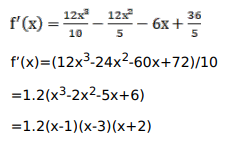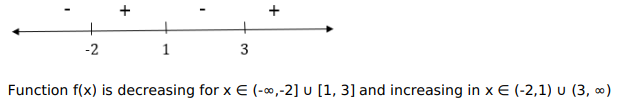# Find the intervals on which each of the following functions is

Question:

Find the intervals on which each of the following functions is (a) increasing (b) decreasing.

$f(x)=\frac{3}{10} x^{4}-\frac{4}{5} x^{3}-3 x^{2}+\frac{36}{5} x+11$

Solution: